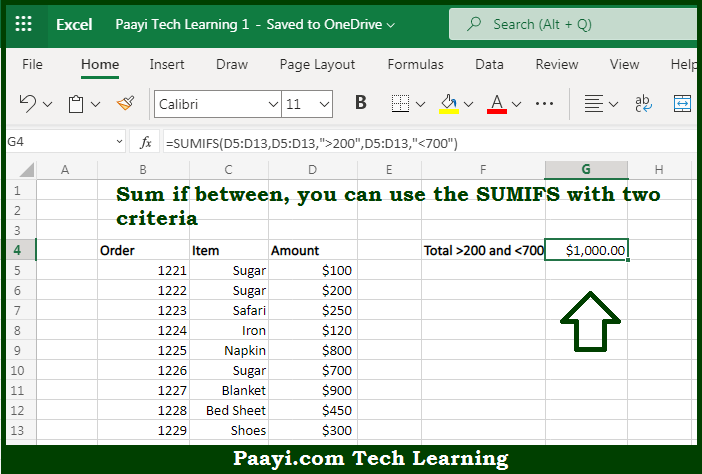# Learn How to SUM If Between in Microsoft Excel

Written by | 0 Comments | 519 Views

In this article, you will learn how to SUM various things in Microsoft Excel using a single/combination(s) of functions. You will also know how to SUM If Between and see the generic formula.

SUM If Between in Microsoft Excel

The main purpose of this formula is to sum if between the two values. Here we will learn how to sum if between the specific value in the workbook in Microsoft Excel. That implies, with the help of a formula based on the SUMIFS function you can able to sum if between the two values. So, with the help of this formula, you can able sum if between the specific value in the workbook in Microsoft Excel.

General Formula to SUM If Between

=SUMIFS(sum_range,criteria_range,">500",criteria_range,"<1000")

The Explanation for the SUM If BetweenSo we know that with the help of the given formula above you can able sum if between the two values. Here we will learn how to sum if between the specific value in the workbook in Microsoft Excel. As we know that the SUMIFS function supports logical operators in Microsoft Excel like - "=",">",">=", etc. So you can use these operators as per your criteria in the formula. It should be noted that both operators (>, <) and threshold amounts are enclosed in double quotes (" "). So, with the help of this formula, you can able to sum if between the specific value in the workbook in Microsoft Excel.